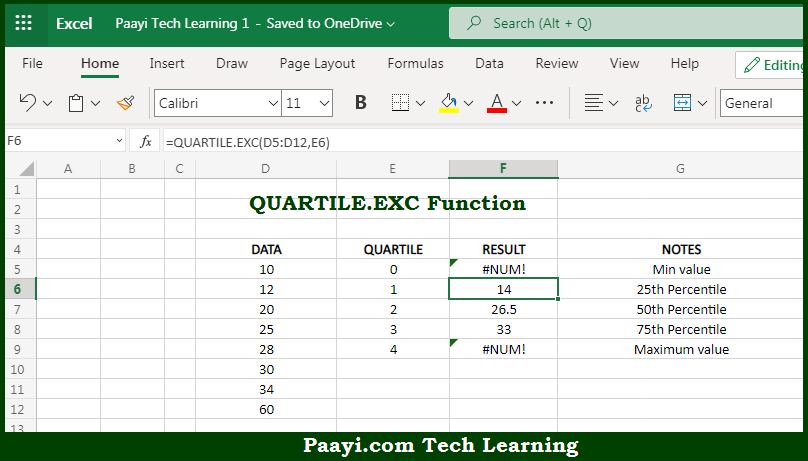# Learn How to Use Microsoft Excel QUARTILE.EXC Function

Written by | 0 Comments | 617 Views

In this article, you will learn how to use Microsoft Excel QUARTILE.EXC function and its prime function in Microsoft Excel. You will also get to know the Microsoft Excel QUARTILE.EXC function return value and syntax with the help of some examples.

Microsoft Excel QUARTILE.EXC Function

The main purpose of the Microsoft Excel QUARTILE.EXC function is to get the quartile in the given data set. That implies, with the help of QUARTILE.EXC function you can able to return the quartile in a given set of data. It should be noted that the QUARTILE.EXC function can return, first quartile, second quartile, and third quartile. So, with the help of QUARTILE.EXC function, you can able to get the quartile in the given data set.

Return Value of QUARTILE.EXC Function

The return value will be the value for the requested quartile.

Syntax of QUARTILE.EXC Function

=QUARTILE.EXC(array, quart)

Where the arguments:

• array: This is the reference containing data to analyze.
• quart: This is the quartile value to return, 1 to 3.

## How to Use Microsoft Excel QUARTILE.EXC Function?So we know that, Microsoft Excel QUARTILE.EXC function you can able to get the quartile in the given data set. That implies, with the help of QUARTILE.EXC function you can able to return the quartile in a given set of data. It should be noted that the QUARTILE.EXC function can return, first quartile, second quartile, and third quartile. So, with the help of QUARTILE.EXC function, you can able to get the quartile in the given data set.